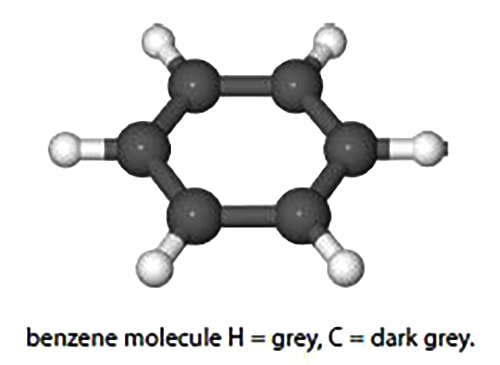Chemistry

# Enthalpy of Molecule and Temperature Changes with Integration

The enthalpy of a molecule, H, changes by dH when the temperature, T, changes by dT and the change is commonly given as a polynomial equation

dH = (a + bT + -cT2) dT

Calculate the change in the enthalpy, dH, of benzene (below Fig) when it is heated from 298 K to 398 K by integrating the polynomial given the following constants for benzene.

a = – 1.70 J mo1-1 K-1

b = 3.25 x 10-1 J mol-1 K-2

c = 1.11 x 10-4 J mo1-1 K-3So,

Integrating from T1 to T2.For clarity of presentation, below I have split the evaluation of the integrated equation over two linesΔ H = 1.249 x 104 J mol-1

Δ H = 12.49 kJ mol-1

As a final step the enthalpy change of the benzene upon heating has been quoted in the conventional units of kJ mol-1.